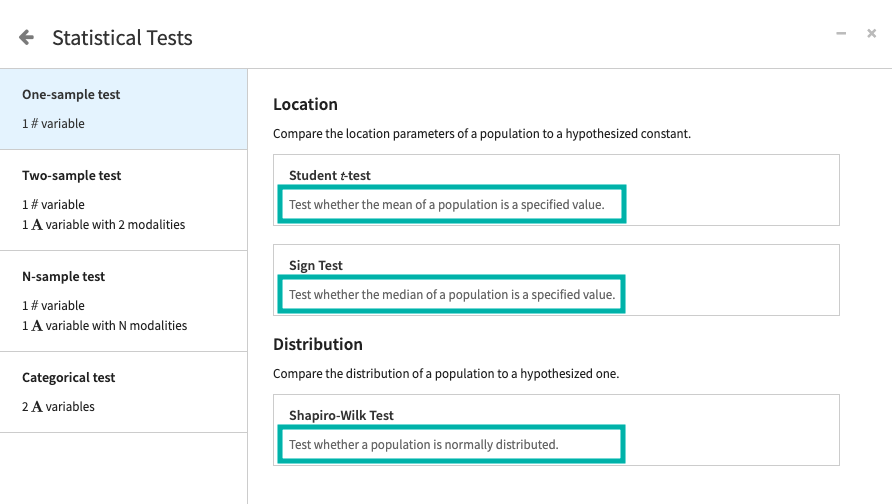# Concept: Hypothesis Testing¶

Recall that a hypothesis test allows you to evaluate a pair of competing hypotheses (the null and alternative hypotheses) about the properties of a population, such as its distribution or parameters of the distribution.

Hypothesis test cards in Dataiku DSS display the purpose of the test, to guide in choosing the correct test for our use case.To create a card, specify one or more test variables, and in some cases, specify additional parameters.

Dataiku DSS uses an alpha value (or significance level) of 0.05 to determine how rare an event must be for us to reject the null hypothesis. DSS also computes an appropriate test statistic value and calculates the p value. By comparing the p value and significance level, DSS can arrive at one of these conclusions:

• Reject the null hypothesis, if $$p \leq alpha$$

• Determine that the test is inconclusive, if $$p > alpha$$

The header of any hypothesis test card includes a question icon that provides detailed information about the test, such as: what kind of test it is, the underlying assumptions for the test to be meaningful, and so on.